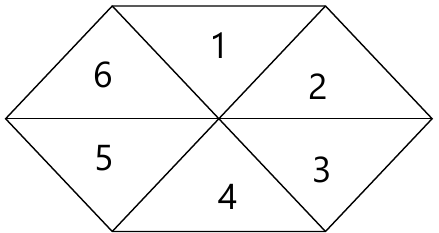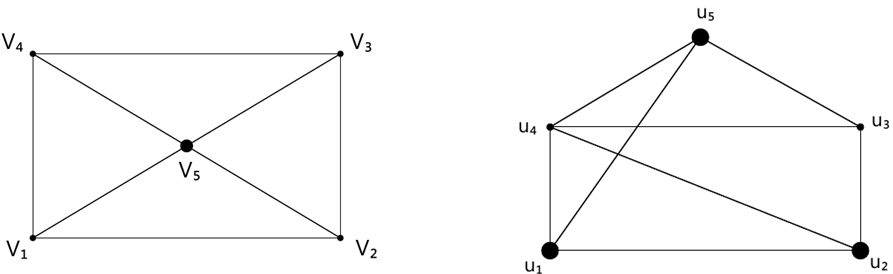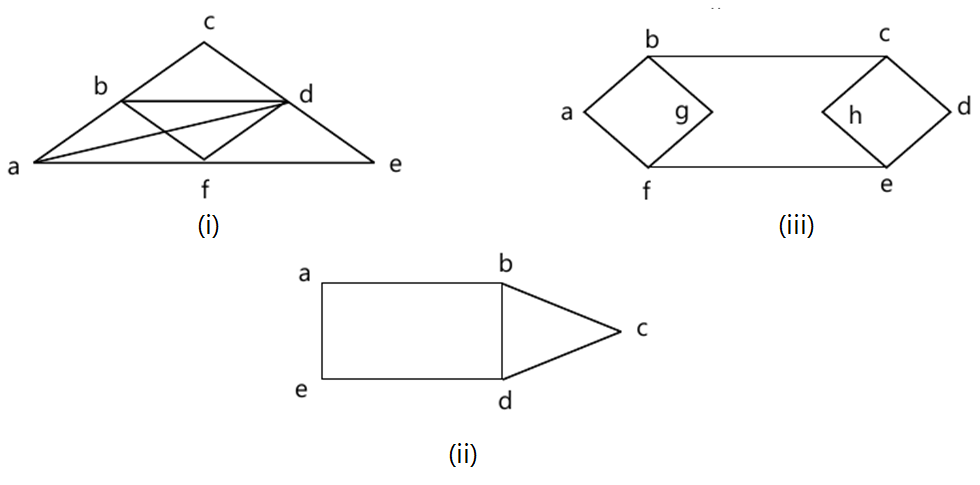MORE IN Discrete Structures
MU Computer Engineering (Semester 3)
Discrete Structures
May 2013
Total marks: --
Total time: --
INSTRUCTIONS
(1) Assume appropriate data and state your reasons
(2) Marks are given to the right of every question
(3) Draw neat diagrams wherever necessary

1 (a) Prove by Mathematical Induction -
$1^2+2^2+3^2+\bullet{}\bullet{}\bullet{}\bullet{}\bullet{}\bullet{}\bullet{}\bullet{}n^2=\frac{n(n+1)(2n+1)}{6}$
8 M
1 (b) Explain the terms :-
(i) Poset
(ii) Normal Subgroup
(iii) Lattice.
6 M
1 (c) In a survey of 60 people, it was found that 25 reads Newsweek Magazine, 26 reads Times and 26 reads Fortune. Also 9 reads both Newsweek and Fortune, 11 reads both Newsweek and Times, 8 reads Times and Fortune and 8 reads no magazine at all.
(i) Find the number of people who read all three magazines.
(ii) Determine number of people who read exactly one magazine.
6 M

2 (a) Determine injective, surjective and bijective functions. If f: R → R and g: R → R are defined by the formulas - f(x)=x+2 and g(x)=x2 find (i) f.g.f. (ii) g.f.g.
8 M
2 (b) Define equivalence relation on a set. Let R be a relation on the set of integers defined by a Rb iff a-b is a multiple of 5. prove that R is a equivalence relation.
6 M
2 (c) State the converse, inverse and contrapositive of the following :-
(i) If it is cold, then he wears a hat.
(ii) If an integer is a multiple of 2, then it is even.
6 M

Explain Hasse diagram. Draw the Hasse diagram of the relation given by
3 (a)(i) R1= { (1,1), (1,2), (1,3), (1,4), (1,5), (2,2), (2,3), (2,4), (2,5), (3,3), (3,4), (3,5), (4,4), (4,5), (5,5) }
4 M
3 (a)(ii) R2= { (1,1), (1,3), (1,4), (1,5), (2,2), (2,3), (2,4), (2,5), (3,3), (3,4), (3,5), (4,4), (5,5), }
4 M
3 (b) Let A= { 1, 2, 3 4} and R= { (1,2), (2,3), (3,4), (2,1)}. Find the Transitive closure of R using Warshall's Algorithm.
6 M
3 (c) Consider the region shown below. It is bounded by a regular hexagon whose sides are the length 1 units. Show that if any seven points are chosen in this region then two of them must be no further apart than 1 unit.6 M

4 (a) Show that the following graphs are isomorphic. :8 M
4 (b) Let R={ (1,2), (4,3), (2,2), (2,1), (3,1) } be a relation on s={1,2,3,4}. Find the symmetric closure of R.
6 M
4 (c) Define :-
(i) Interal domain
(ii) Field
(iii) Normal Subgroup.
6 M

5 (a) What is a minimum spanning tree? Explain one techniques with example.
8 M
5 (b) Define Cyclic Group. Prove that the set A = { 0, 1, 2, 3, 4, 5} is a finite abelian under addition modulo 6.
6 M
5 (c) Determine whether the given graph has a Hamilton circuit or Eulerian circuit. If it does, find such a circuit :6 M

More question papers from Discrete Structures